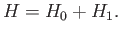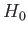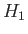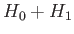Next: Two-State System Up: Time-Independent Perturbation Theory Previous: Time-Independent Perturbation Theory

# Introduction

We have developed techniques by which the general energy eigenvalue problem can be reduced to a set of coupled partial differential equations involving various wavefunctions. Unfortunately, the number of such problems that yield exactly soluble systems of equations is comparatively small. It is, therefore, necessary to develop techniques for finding approximate solutions to otherwise intractable problems.

Consider the following problem, which is very common. The Hamiltonian of some quantum mechanical system is written(7.1)

Here,is a simple Hamiltonian for which we know the exact eigenvalues and eigenstates.introduces some interesting additional physics into the problem, but it is sufficiently complicated that, when we add it to, we can no longer find the exact energy eigenvalues and eigenstates. However,can, in some sense (which we shall specify more exactly later on), be regarded as small compared to. Let us try to find approximate eigenvalues and eigenstates of the modified Hamiltonian,, by performing a perturbation expansion about the eigenvalues and eigenstates of the original Hamiltonian,.

We shall start, in this chapter, by considering time-independent perturbation theory , in which the modification to the Hamiltonian,, has no explicit dependence on time. It is usually assumed that the unperturbed Hamiltonian,, is also time independent.Next: Two-State System Up: Time-Independent Perturbation Theory Previous: Time-Independent Perturbation Theory
Richard Fitzpatrick 2016-01-22